# Chapter 12 Lateral and Surface Areas 12 2

• Slides: 32Chapter 12 Lateral and Surface Areas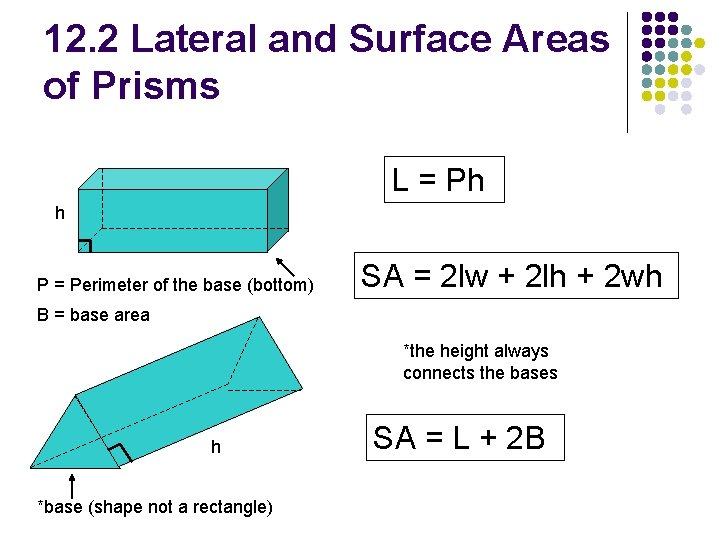12. 2 Lateral and Surface Areas of Prisms L = Ph h P = Perimeter of the base (bottom) SA = 2 lw + 2 lh + 2 wh B = base area *the height always connects the bases h *base (shape not a rectangle) SA = L + 2 B12. 2 Lateral and Surface Areas of Prisms Find the lateral and surface areas of the rectangular prism.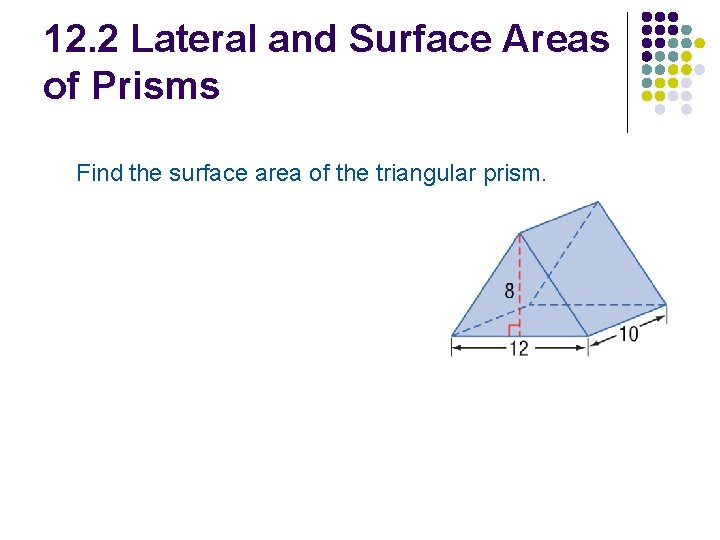12. 2 Lateral and Surface Areas of Prisms Find the surface area of the triangular prism.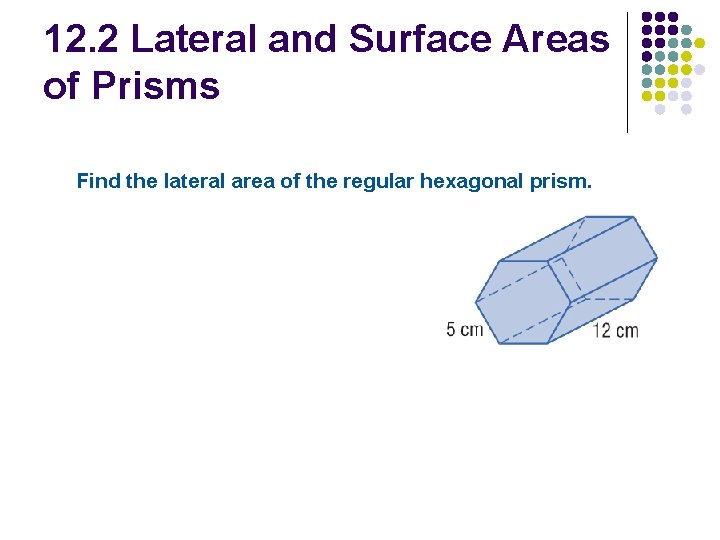12. 2 Lateral and Surface Areas of Prisms Find the lateral area of the regular hexagonal prism.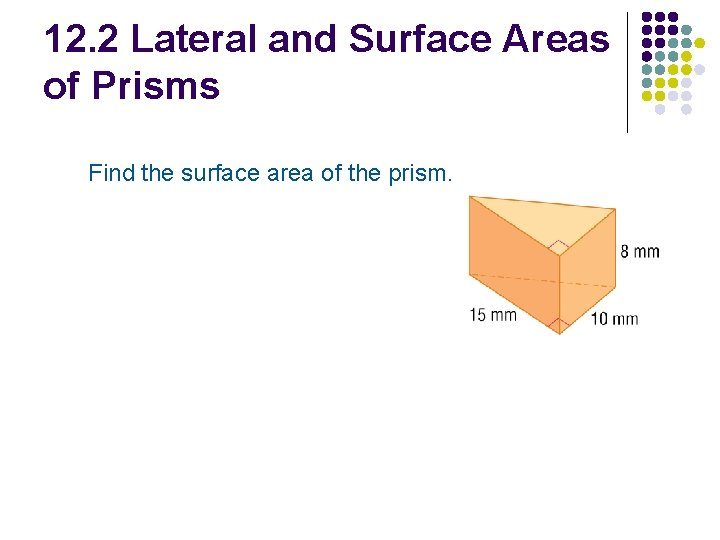12. 2 Lateral and Surface Areas of Prisms Find the surface area of the prism.12. 2 Lateral and Surface Areas of Prisms Find the lateral area of the prism.12. 2 Lateral and Surface Areas of Prisms The lateral area of a prism is 476 square meters. The area of one of its bases is 25 square meters. What is the surface area of the prism?12. 3 Lateral and Surface Area of Cylinders r L = 2πrh h S = 2πrh + 2πr 2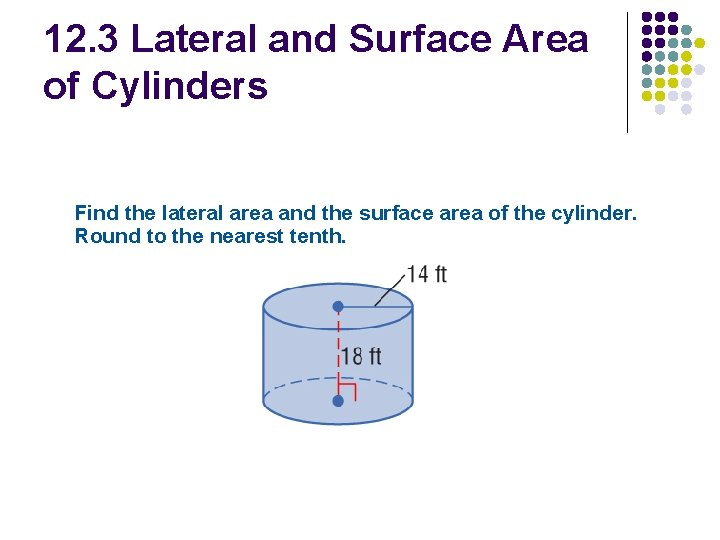12. 3 Lateral and Surface Area of Cylinders Find the lateral area and the surface area of the cylinder. Round to the nearest tenth.12. 3 Lateral and Surface Area of Cylinders Find the lateral area and the surface area of the cylinder. Round to the nearest tenth.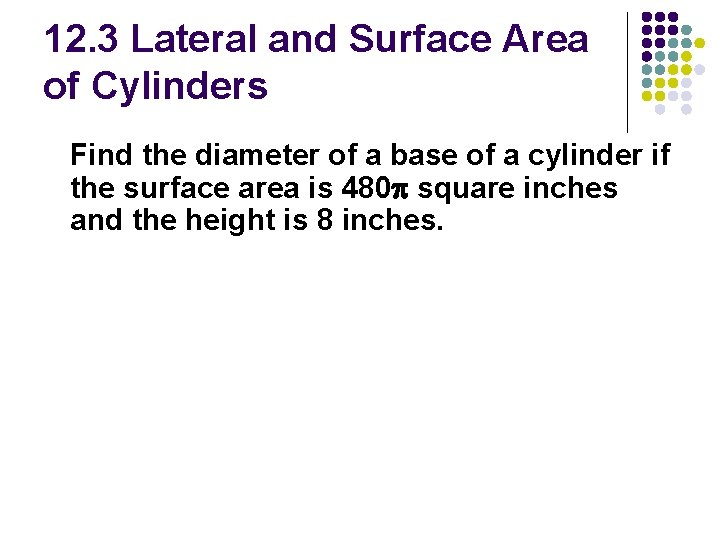12. 3 Lateral and Surface Area of Cylinders Find the diameter of a base of a cylinder if the surface area is 480 square inches and the height is 8 inches.12. 4 Lateral and Surface Areas of Pyramids h l h L= l S= = slant height l ½b Lateral edge l ½b Pl Pl + B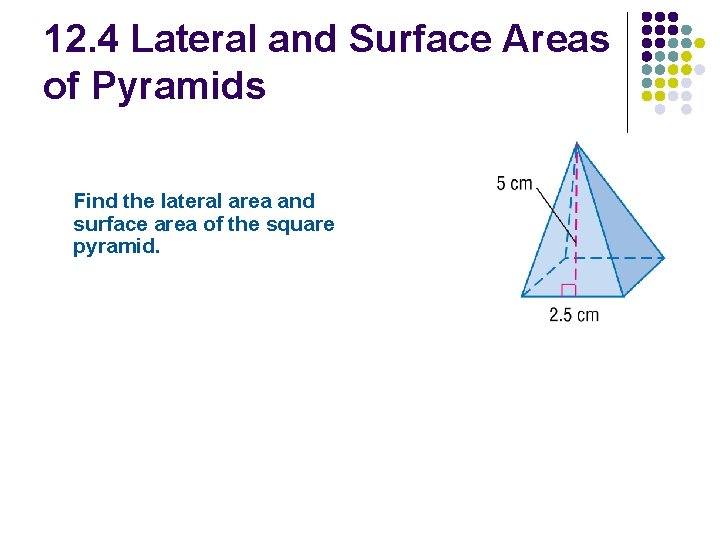12. 4 Lateral and Surface Areas of Pyramids Find the lateral area and surface area of the square pyramid.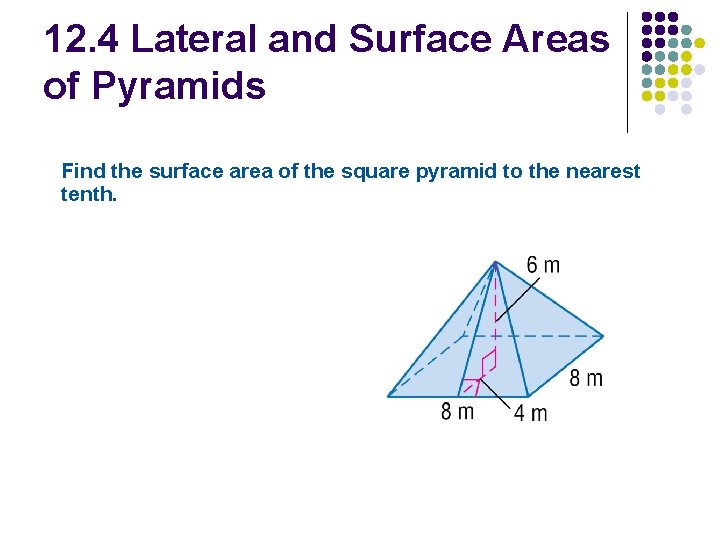12. 4 Lateral and Surface Areas of Pyramids Find the surface area of the square pyramid to the nearest tenth.12. 4 Lateral and Surface Areas of Pyramids Find the lateral and surface areas of the regular pyramid. Round to the nearest tenth.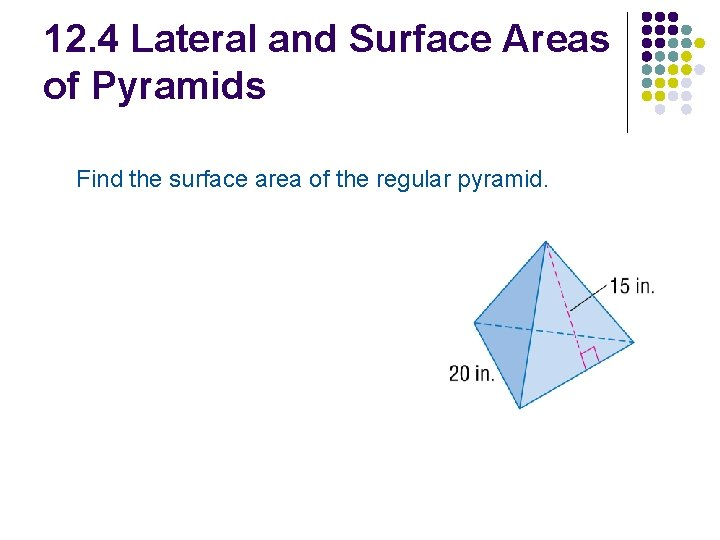12. 4 Lateral and Surface Areas of Pyramids Find the surface area of the regular pyramid.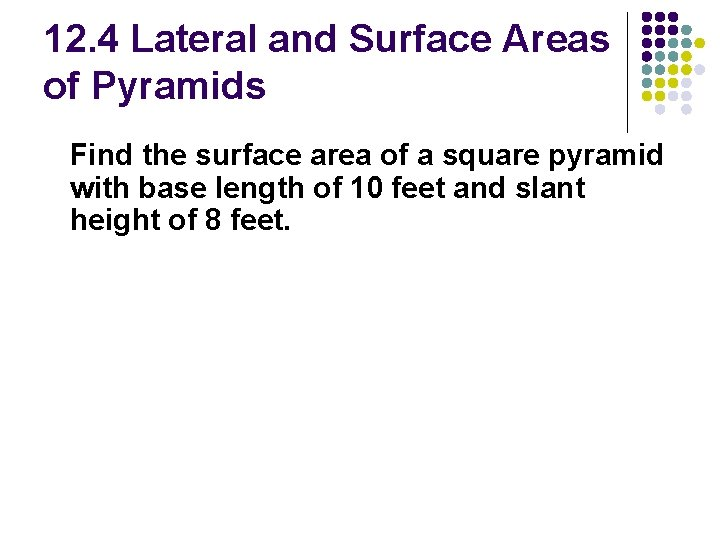12. 4 Lateral and Surface Areas of Pyramids Find the surface area of a square pyramid with base length of 10 feet and slant height of 8 feet.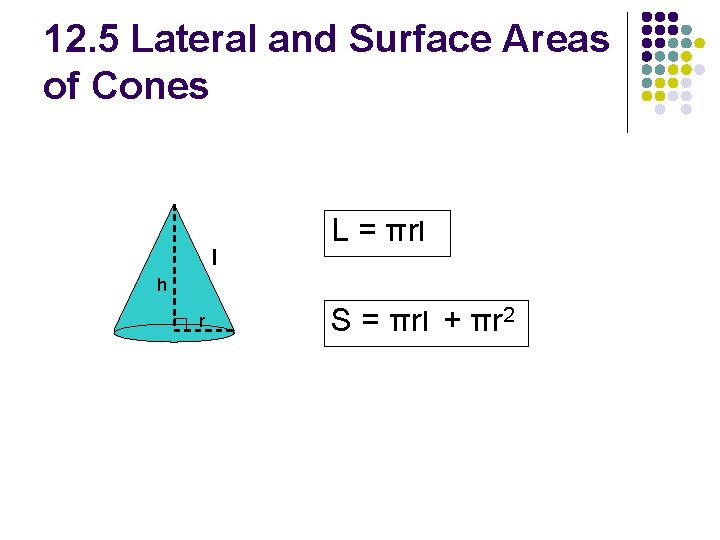12. 5 Lateral and Surface Areas of Cones l L = πrl h r S = πrl + πr 2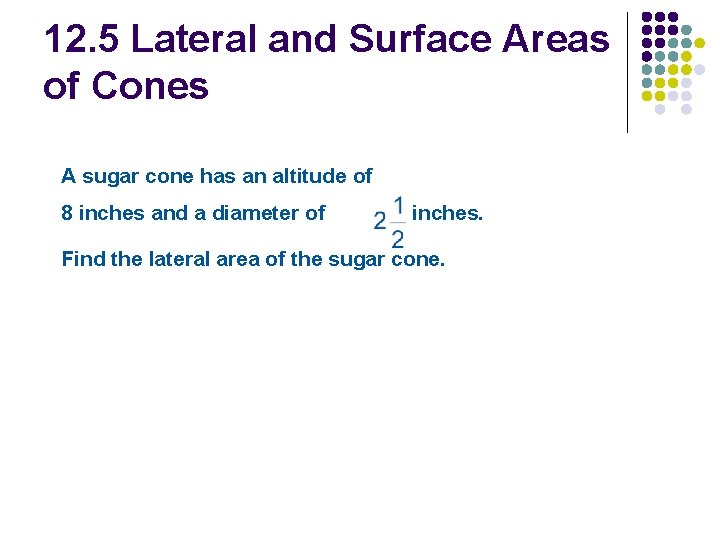12. 5 Lateral and Surface Areas of Cones A sugar cone has an altitude of 8 inches and a diameter of inches. Find the lateral area of the sugar cone.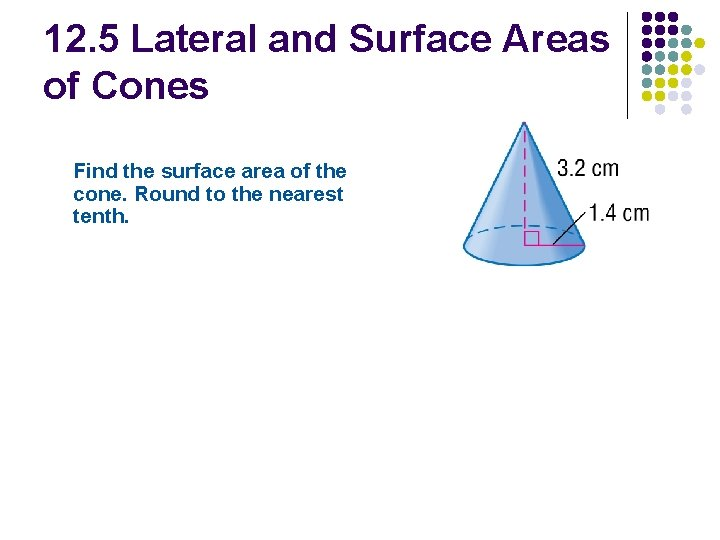12. 5 Lateral and Surface Areas of Cones Find the surface area of the cone. Round to the nearest tenth.12. 5 Lateral and Surface Areas of Cones Find the surface area of the cone.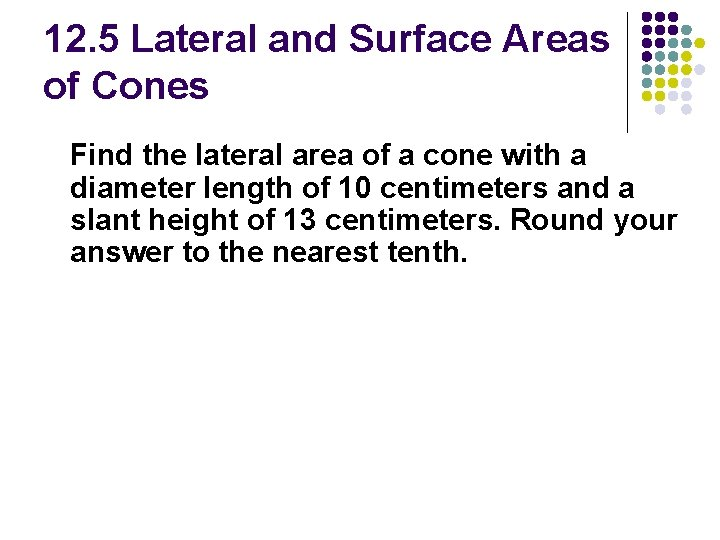12. 5 Lateral and Surface Areas of Cones Find the lateral area of a cone with a diameter length of 10 centimeters and a slant height of 13 centimeters. Round your answer to the nearest tenth.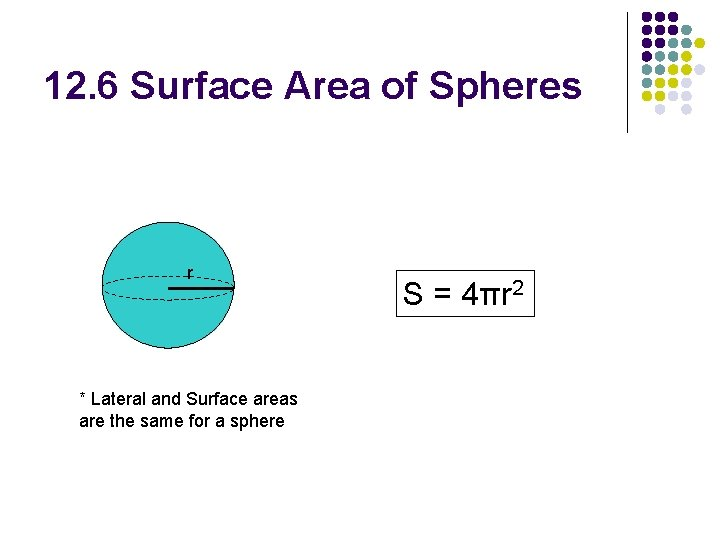12. 6 Surface Area of Spheres r * Lateral and Surface areas are the same for a sphere S = 4πr 212. 6 Surface Area of Spheres Find the surface area of the sphere. Round to the nearest tenth.12. 6 Surface Area of Spheres Find the surface area of the sphere. Round to the nearest tenth.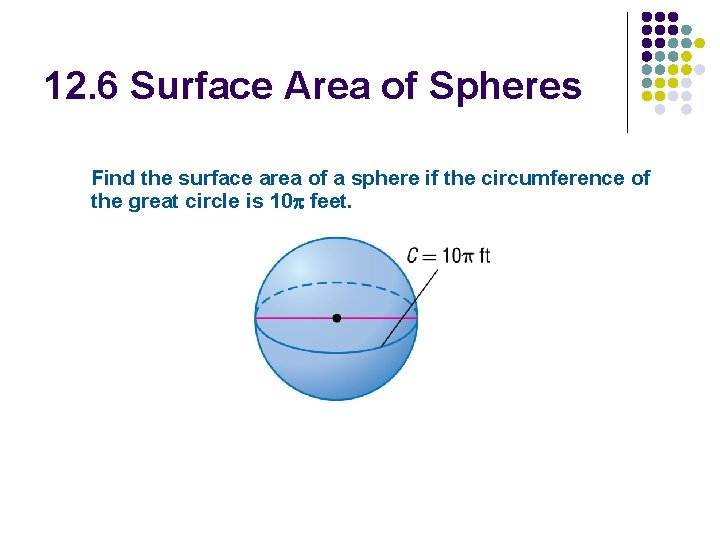12. 6 Surface Area of Spheres Find the surface area of a sphere if the circumference of the great circle is 10 feet.12. 6 Surface Area of Spheres Find the surface area of a sphere if the area of the great circle is approximately 220 square meters.12. 6 Surface Area of Hemispheres S = ½ 4πr 2 + πr 2 r or S = 3πr 212. 6 Surface Area of Spheres Find the surface area of the hemisphere.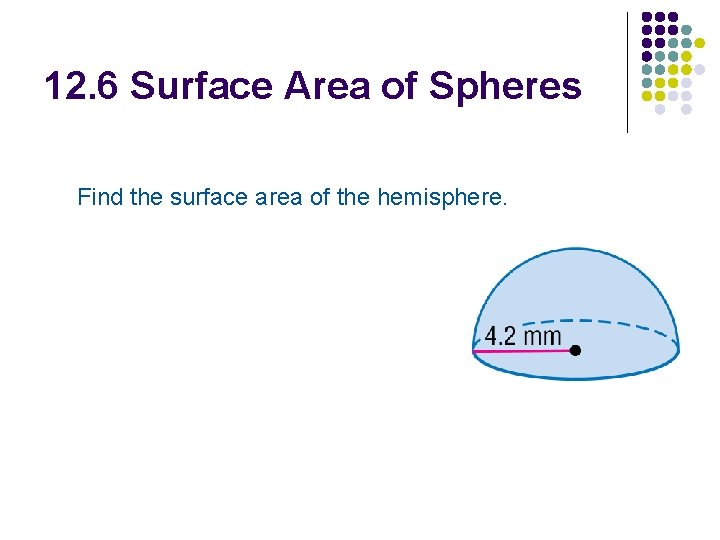12. 6 Surface Area of Spheres Find the surface area of the hemisphere.12. 6 Surface Area of Spheres A hemisphere has a giant circle with circumference approximately 175. 84 in 2. What is the surface area of the hemisphere?